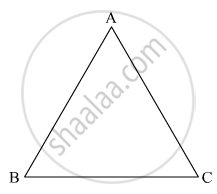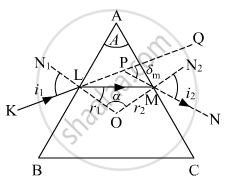Department of Pre-University Education, KarnatakaPUC Karnataka Science Class 12

# A Ray of Light Passing from Air Through an Equilateral Glass Prism Undergoes Minimum Deviation When the Angle of Incidence Is 3/4 Th Of the Angle of Prism. Calculate the Speed of Light in the Prism. - Physics

A ray of light passing from air through an equilateral glass prism undergoes minimum deviation when the angle of incidence is 3/4 th of the angle of prism. Calculate the speed of light in the prism.

A ray of light incident on face AB of an equilateral glass prism, shows minimum deviation of 30°. Calculate the speed of light through the prism.#### Solutioni = 3/4 A for minimum deviation we know,
A + δm = 2i

Therefore, A + δm = 2 × 3/4 A

δm = (1.5 − 1)A

⇒ δm = 0.5 A

For equilateral prism

A = 60° ...(i)

⇒ δm = 0.5 × 60° = 30° ...(ii)

Also, for minimum deviation, refractive index of glass w.r.t. air is given by

∴μ="speed of light in air (c)"/"speed of light in prism"...(iii)

thereforemu=sin((A+δ_m)/2)/sin(A/2)

∴μ=sin((60+30)^@/2)/sin(60^@/2)

∴μ=sin((90^@)/2)/sin(60^@/2)   ....(iv)

using  (iii) and (iv)

∴"speed of light in prism"="speed of light in air (c)"/μ

∴"speed of light in prism" =sin(60^@/2)/sin(90^@/2) xx c

= (1/2)/(1/sqrt2)xx  3 × 10^8

= 3/sqrt2 × 10^8 "m/s"

= 2.12 × 10^8 "m/s"

Concept: Refraction Through a Prism
Is there an error in this question or solution?